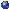## Events

##### TopologyMehdi Yazdi, Zoom: The complexity of determining knot genus in a fixed 3-manifold
Monday, April 05, 2021, 02:00pm - 03:00pm
The genus of a knot in a 3-manifold is defined to be the minimum genus of a compact, orientable surface bounding that knot, if such a surface exists. In particular a knot can be untangled if and only if it has genus zero. We consider the computational complexity of determining knot genus. Such problems have been studied by several mathematicians; among them are the works of Hass-Lagarias-Pippenger, Agol-Hass-Thurston, Agol, and Lackenby. For a fixed 3-manifold the knot genus problem asks, given a knot K and an integer g, whether the genus of K is equal to g. Marc Lackenby proved that the knot genus problem for the 3-sphere lies in NP. In joint work with Lackenby, we prove that this can be generalized to any fixed, compact, orientable 3-manifold, answering a question of Agol-Hass-Thurston from 2002.Location: Zoom